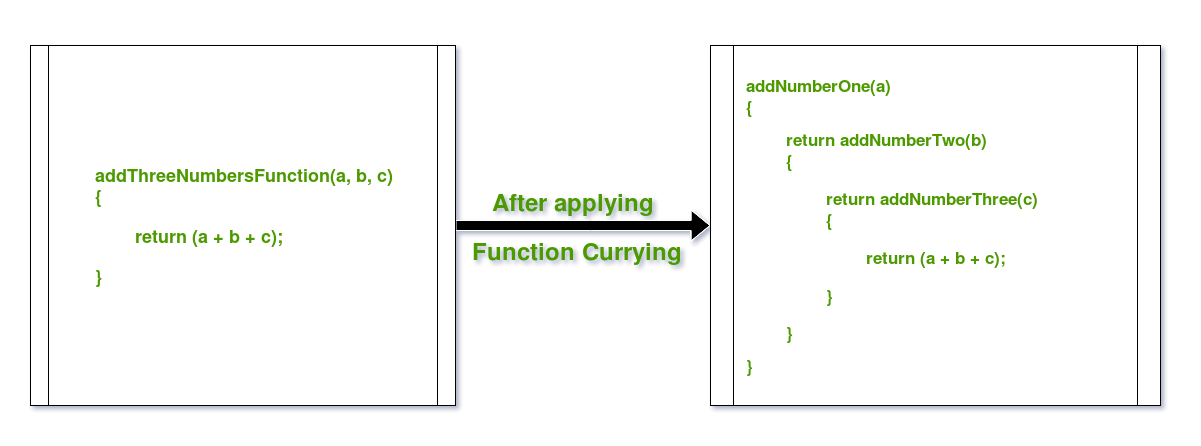# Currying Functions in Java with Examples

• Difficulty Level : Medium
• Last Updated : 18 Sep, 2018

Function Currying is a concept of breaking a function with many arguments into many functions with single argument in such a way, that the output is same. In other words, its a technique of simplifying a multi-valued argument function into single-valued argument multi-functions.

Consider the example to clear the concept:Currying breaks down higher order functions into a series of smaller cascaded functions which take in one argument and return a function except for the last cascaded function which returns the desired value.

For example:

Let there be a function which maps asCurrying the above function will produceThusmaps fromto a function which in turn maps fromtoThe above mathematical expression can also be represented as:Hence,Below are some examples in Java to demonstrate Function Currying:

Example 1: Adding 2 numbers using Function Currying

 `// Java Program to demonstrate Function Currying ` ` `  `import` `java.util.function.Function; ` ` `  `public` `class` `GFG { ` `    ``public` `static` `void` `main(String args[]) ` `    ``{ ` ` `  `        ``// Using Java 8 Functions ` `        ``// to create lambda expressions for functions ` `        ``// and with this, applying Function Currying ` ` `  `        ``// Curried Function for Adding u & v ` `        ``Function > ` `            ``curryAdder = u -> v -> u + v; ` ` `  `        ``// Calling the curried functions ` ` `  `        ``// Calling Curried Function for Adding u & v ` `        ``System.out.println(``"Add 2, 3 :"` `                           ``+ curryAdder ` `                                 ``.apply(``2``) ` `                                 ``.apply(``3``)); ` ` `  `        ``} ` `} `

Output:

```Add 2, 3 :5
```

Example 2: Multiplying 2 numbers using Function Currying

 `// Java Program to demonstrate Function Currying ` ` `  `import` `java.util.function.Function; ` ` `  `public` `class` `GFG { ` `    ``public` `static` `void` `main(String args[]) ` `    ``{ ` ` `  `        ``// Using Java 8 Functions ` `        ``// to create lambda expressions for functions ` `        ``// and with this, applying Function Currying ` ` `  `        ``// Curried Function for Multiplying u & v ` `        ``Function > ` `            ``curryMulti = u -> v -> u * v; ` ` `  `        ``// Calling the curried functions ` `        `  `        ``// Calling Curried Function for Multiplying u & v ` `        ``System.out.println(``"Multiply 2, 3 :"` `                           ``+ curryMulti ` `                                 ``.apply(``2``) ` `                                 ``.apply(``3``)); ` `    ``} ` `} `

Output:

```Multiply 2, 3 :6
```

Example 3: Adding 3 numbers using Function Currying

 `// Java Program to demonstrate Function Currying ` ` `  `import` `java.util.function.Function; ` ` `  `public` `class` `GFG { ` `    ``public` `static` `void` `main(String args[]) ` `    ``{ ` ` `  `        ``// Using Java 8 Functions ` `        ``// to create lambda expressions for functions ` `        ``// and with this, applying Function Currying ` ` `  `        ``// Curried Function for Adding u, v & w ` `        ``Function > > ` `            ``triadder = u -> w -> v -> u + w + v; ` ` `  `        ``// Calling the curried functions ` ` `  `        ``// Calling Curried Function for Adding u, v & w ` `        ``System.out.println(``"Add 2, 3, 4 :"` `                           ``+ triadder ` `                                 ``.apply(``2``) ` `                                 ``.apply(``3``) ` `                                 ``.apply(``4``)); ` `    ``} ` `} `

Output:

```Add 2, 3, 4 :9
```

My Personal Notes arrow_drop_up
Recommended Articles
Page :